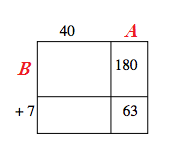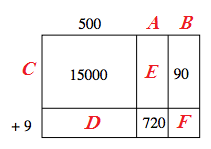Home > CC1 > Chapter 8 > Lesson 8.1.2 > Problem8-29

8-29.

Solve each generic-rectangle puzzle. Write your answer in the form (total length)(total width) = sum of individual area parts = total area. Homework Help ✎

1.  $40$ $180$ $+7$ $63$

In order to solve this rectangle, it might be useful to find the length of side A first. Do this using the known information, 7 times A is equal to 63. Can you find what A equals with division?

If A equals 9, can you find what side B is? Use division and the knowledge that A (9) times B is equal to 180.

$\frac{180}{9}\;=\;\textit{B}$

Once you find that B is equal to 20, you know that the total length equals 49 and the total width equals 27.
Can you fill in the rest of the rectangle and write your answer in the form originally provided in the instructions?1.  $500$ $15000$ $90$ $+9$ $720$

• Using the same strategy as part (a), find the length of sides A, B, and C. With that knowledge, you will be able to find the values of D, E, and F.

It would help if you found A or C first. Remember, in the final steps, these values will serve in the tens place value.

$A = 80, B = 3, \text{ and } C = 30.$

$(583)(39) = 15000 + 4500 + 2400 + 720 + 90 + 27 = 22737$